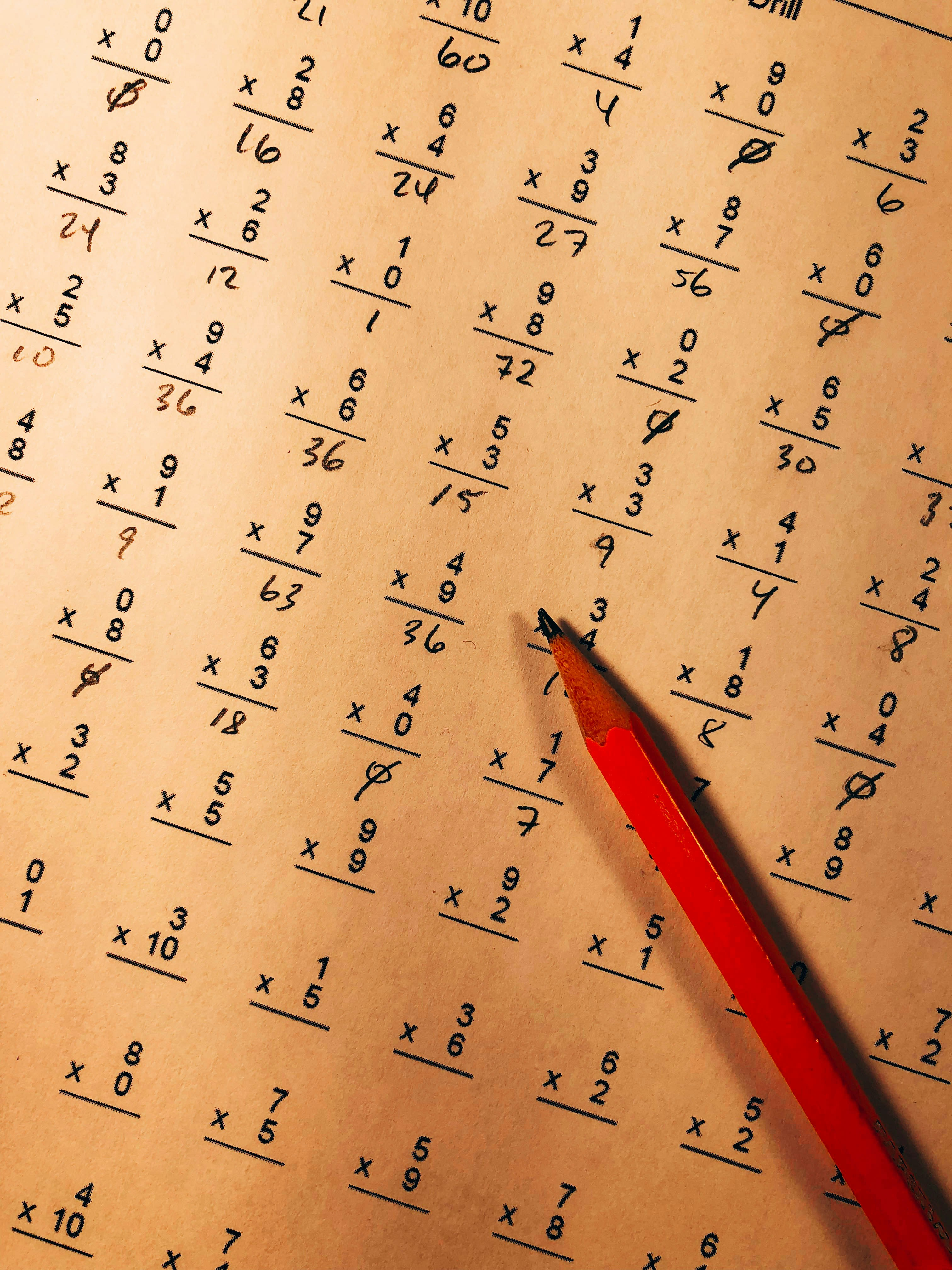Hackerrank - Between Two Sets Solution

# Hackerrank - Between Two Sets SolutionYou will be given two arrays of integers and asked to determine all integers that satisfy the following two conditions:

1. The elements of the first array are all factors of the integer being considered
2. The integer being considered is a factor of all elements of the second array

These numbers are referred to as being between the two arrays. You must determine how many such numbers exist.

For example, given the arrays  and , there are two numbers between them:  and . , ,  and  for the first value. Similarly, ,  and , .

Function Description

Complete the getTotalX function in the editor below. It should return the number of integers that are betwen the sets.

getTotalX has the following parameter(s):

• a: an array of integers
• b: an array of integers

Input Format

The first line contains two space-separated integers,  and , the number of elements in array  and the number of elements in array .
The second line contains  distinct space-separated integers describing  where .
The third line contains  distinct space-separated integers describing  where .

Constraints

Output Format

Print the number of integers that are considered to be between  and .

Sample Input

2 3
2 4
16 32 96


Sample Output

3


Explanation

2 and 4 divide evenly into 4, 8, 12 and 16.
4, 8 and 16 divide evenly into 16, 32, 96.

4, 8 and 16 are the only three numbers for which each element of a is a factor and each is a factor of all elements of b.

### Solution in Python

from math import gcd

def get_hcf(arr):
hcf = arr
for i in arr:
hcf = gcd(hcf,i)
return hcf

def lcm(a,b):
return a*b//gcd(a,b)

def get_lcm(arr):
l = arr
for i in arr:
l = lcm(l,i)
return l

def getTotalX(a,b):
lcm_a = get_lcm(a)
hcf_b = get_hcf(b)
no_between_sets = sum(1 for i in range(lcm_a, hcf_b+1, lcm_a) if not hcf_b%i and i%lcm_a==0)
return no_between_sets

n,m = map(int,input().split())
a = list(map(int,input().split()))
b = list(map(int,input().split()))

print(getTotalX(a,b))

• Numbers must be between LCM of a and HCF of b
• Numbers must be a divisor of HCF of b
• Numbers must be divisible by LCM of a

All the numbers between the lcm of a and the hcf of b that divides hcf of b  and is divisible by lcm of a, are the required numbers.